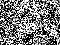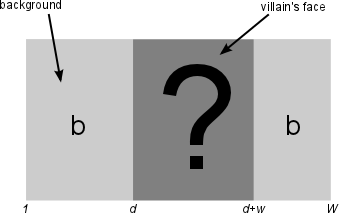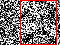Warning

# The EM Algorithm for Nick Carter

Famed investigator Nick Carter got into trouble when solving the case of the mysterious disappearance of lady Thuns's dog. The only clue he was able to obtain was a set of photographs of the likely villain. Unfortunately, the villain is nearly indecipherable in the photographs. Nick is just a detective, so he needs your help to recover the image of the villain from the corrupted photos. He has watched too many episodes of CSI and thinks it can be done. Can it?

## The Clues

So the technically challenged Nick has $n$ corrupted images of $H \times W$ pixels (Figure 2). He knows that each image depicts the face of the villain and that the faces occupy a subwindow in each of the images. Specifically, the image size of a face is only $H \times w$ pixels, i.e, the face spans the height of the image but is narrower than the image. Thus only the horizontal position of the face varies in the images (i.e. we can assume that horizontal position $d$ is the only unknown). Nick also knows that the background of the image, the image area outside the face itself, has constant intensity $b$. See Figure 3. The images are very noisy (assume Gaussian noise distribution here).Figure 2. One of the Nick's imagesFigure 3. Image composition. The face is at an unknown horizontal position $d$.

## The Model

You are given set of $n$ noisy images each with dimension $H \times W$ such that each image contains the same hidden face. The face is of fixed width $w = 36$ pixels and has height equal to its containing image $H$, but in each image it is translated by some unknown and different horizontal displacement. The challenge is to reconstruct the “most likely” image of the face from all the noisy views. “Most likely” can be directly interpreted as the maximum likelihood image of the face as will be shown below.

Assume the following model generated the image set $X = \{x_1,x_2,\ldots,x_n\}$ (i.e., the images that contain the hidden face):

• The background of the image (the part of the image not occupied by the face) is of fixed but unknown intensity $b$.
• The uncorrupted image of the face in image $x_k$, denote it $f$, is moved by some non-negative horizontal displacement $d_k$ relative to the left side of the image $x_k$.
• The entire image is then corrupted by zero mean Gaussian noise with variance $\sigma^2$.

## Supervised Context

For easier notation in the following derivation, let's group the parameters we wish to estimate as $\theta = (f,b,\sigma)$. Then the likelihood $\Lambda_c$ (the subscript c is used for complete data, i.e., both images and displacements are known) of observing image set $X =\{x_1,x_2,\ldots,x_n\}$ is \begin{align*} \Lambda_c(X,\{d_k\}_{k=1}^n;\theta) &= \prod_k P_{X,D}(x_k,d_k;\theta) \\ &= \prod_k P_{X \mid D}(x_k \mid d_k;\theta)P_{D}(d_k;\theta) \end{align*} and note that if we're in the supervised learning case, i.e., if we are given the images AND the locations of the face, we can directly maximize the log likelihood \begin{align*} \theta^* &= \arg\max_{\theta} \Lambda_c(X,\{d_k\}_{k=1}^n;\theta) \\ &= \arg\max_{\theta} \prod_k P_{X \mid D}(x_k \mid d_k;\theta)P_{D}(d_k;\theta) \\ &= \arg\max_{\theta} \sum_k \log\left( P_{X \mid D}(x_k \mid d_k;\theta)P_{D}(d_k;\theta) \right) \\ &= \arg\max_{\theta} \lambda_c(X,\{d_k\}_{k=1}^n;\theta). \end{align*} Since each of the background and face is corrupted by Gaussian noise, let's introduce some shorthand for the Gaussian so that the forthcoming discussion has less cumbersome notation: $$g(x;\mu,\sigma) = \frac{1}{\sigma \sqrt{2\pi}}e^{-\frac{(x-\mu)^2}{2\sigma^2}}.$$ Let's explicitly specify the distribution of pixel intensities for an image $x_k$ given that we know where the face is, i.e., $P_{X \mid D}(x_k \mid d_k;\theta)$. We are given that the background and face are corrupted by Gaussian noise with the same variance $\sigma^2$. Let $x_k(i,j),f(i,j)$ be the intensity of the pixel at coordinate $(i,j)$ in the image and face respectively. Then, \begin{equation*} P_{X \mid D}(x_k \mid d_k;\theta) = \prod_{ij}\left\{ \begin{array}{ll} g(x_k(i,j);b,\sigma) & 1 \leq j < d_k \text{ or } d_k+w \leq j \leq W \\ g(x_k(i,j);f(i,j-d_k+1),\sigma) & \text{ otherwise } \end{array} \right. \end{equation*} $P_{D}(\cdot)$ is a probability mass function that gives the prior belief that the face is located at a particular displacement. At the start of the investigation, we have no information about where the face is. So initially we will randomly assign a probability to each possible displacement while ensuring that $P_{D}(\cdot)$ is a probability mass function. It should be clear how to maximize the log likelihood $\lambda_c(X,\{d\}_{i=1}^n;\theta)$ in the supervised learning context from lab 4. Unfortunately we are not given the displacements of the face in any image.

## Unsupervised Context

In the unsupervised learning context it is still possible to find the likelihood of observing image set $X = \{x_1,x_2,\ldots,x_n\}$ by treating $\{d_k\}_{k=1}^n$ as hidden variables and marginalizing over all possible displacements \begin{align*} \Lambda(X;\theta) &= \prod_k P_{X}(x_k;\theta) \\ &= \prod_k \sum_{d} P_{X,D}(x_k,d;\theta) \\ &= \prod_k \sum_{d} P_{X \mid D}(x_k \mid d;\theta)P_{D}(d;\theta). \end{align*} Remember that $d$ assumes the integral values $\{1,\ldots,W-w+1\}$. The marginal log likelihood is written as \begin{align*} \log \Lambda(X;\theta) &= \sum_k \log \sum_{d}P_{X \mid D}(x_k \mid d;\theta)P_{D}(d;\theta) \\ &= \lambda(X;\theta) \end{align*} However, $\lambda(X;\theta)$ is difficult to optimize. Note that there is a summation inside the log, which couples the parameters $\theta$, making $\lambda(X;\theta)$ difficult to maximize. EM iteratively maximizes a concave, easier-to-optimize lower bound for the marginal log likelihood $\lambda(X;\theta)$. A very good exposition and theoretical development on EM can be found here: EM notes of Dellaert (pdf)

EM alternates between estimating the distributions over the hidden variables $\{d_k\}_{k=1}^n$ and the unknown parameter vector $\theta$; i.e., it is a block-coordinate ascent. This sounds similar to k-means; however, instead of finding the best $d_k \in D$ given an estimate $\theta$ at each iteration, EM computes a distribution over the space $D$. Specifically, the E-step makes an estimate of the posteriors $P_{D \mid X}$ of the hidden parameters given the current guess of the model parameters $\theta^{(t)}$, and the M-step maximizes the expected complete log-likelihood, defined as \begin{align*} Q^{(t)}(\theta) &= \sum_{k,d} P_{D|X}(d \mid x_k;\theta^{(t)})\log P_{X,D}(x_k,d;\theta) \\ &= \mathbb{E}_{D|X} \left[\log P_{X,D}({x_1,x_2,\ldots,x_n},d;\theta)\right] \end{align*}

### E-step

$P_{D \mid X}(d \mid x_k;\theta^{(t)})$ is the probability that the villain's face $f$ is located at displacement $d$ given the image $x_k$. Given $\theta^{(t)}$, calculate \begin{align*} P_{D \mid X}(d \mid x_k;\theta^{(t)}) &= \frac{P_{X \mid D}(x_k \mid d;\theta^{(t)})P_{D}(d;\theta^{(t)})}{P_X(x_k;\theta^{(t)})} \\ &= \frac{P_{X \mid D}(x_k \mid d;\theta^{(t)})P_{D}(d;\theta^{(t)})}{\sum_d P_{X \mid D}(x_k \mid d;\theta^{(t)})P_D(d;\theta^{(t)})} \end{align*} for each image $k$ over each possible displacement $d \in \{1\ldots W-w+1\}$. Note that given any image $x_k$ $$\sum_d P_{D \mid X}(d \mid x_k;\theta^{(t)}) = 1.$$ A good way to implement this is to store the probabilities of $P_{D \mid X}$ in a 2D look-up table, e.g., define the Matlab array $$\text{Pdx(d,k)} = P_{D \mid X}(d \mid x_k;\theta^{(t)}).$$ This definition will make the Matlab array column stochastic. If $t=0$, there is no valid estimate $\theta^{(0)}$, so randomly initialize $P_{D \mid X}(d \mid x_k;\theta^{(t)})$ enforcing the constraint that $\text{Pdx(d,k)}$ is column stochastic. To prevent underflow, see the section Posterior Calculation. To calculate the priors on displacement, marginalize the images \begin{align} P_D(d) &= \sum_k P_{X,D}(x_k,d) \\ &= \sum_k P_{D \mid X}(d \mid x_k;\theta^{(t)})P_X(x_k) \\ &= \frac{1}{n}\sum_k P_{D \mid X}(d \mid x_k;\theta^{(t)}) \end{align}

### M-step

Given $P_{D \mid X}(d_k \mid x_k;\theta^{(t)})$, which was estimated in the E step, the likelihood of the parameters of interest $\theta$ can be maximized by solving for $\theta$ such that gradient of $\nabla Q^{(t)}(\theta) = 0$ (and verifying that it is a maximum). Stated as an optimization criterion, the M step is $$\theta^{(t+1)} = \arg\max_{\theta}Q^{(t)}(\theta).$$

The update equations needed to calculate $\theta^{(t+1)}$ that are gotten from the constraint $\nabla Q^{(t)}(\theta) = 0$ are provided for you: \begin{align*} f(i,j) &=\frac{1}{n} \sum_{k=1}^{N} \sum_{d=1}^{W-w+1} P_{D|X}(d \mid x_k ; \theta^{(t)}) x_k(i, d+j-1), \\ b &= \frac{1}{nH(W-w)}\sum_{k,d} P_{D \mid X}(d \mid x_k;\theta^{(t)}) \sum_i\sum_{j \notin \{ d,\ldots,d+w-1\} } x_k(i,j), \\ \sigma^2 &= \frac{1}{nHW}\sum_{k,d} P_{D \mid X}(d \mid x_k;\theta^{(t)})(\Delta f(k,d)+\Delta b(k,d)), \\ \end{align*} where \begin{align*} \Delta f(k,d_k) &= \sum_i\sum_{j \in \{1,\ldots,w\} } (x_k(i,j+d_k-1)-f(i,j))^2, \\ \Delta b(k,d_k) &= \sum_i\sum_{j \notin \{ d_k,\ldots,d_k+w-1\} } (x_k(i,j)-b)^2. \\ \end{align*} For formulas derivation see .

## Stopping Criterion

We will test for the idempotency (as defined by some tolerance) of the the marginal log-likelihood we are maximizing to determine when to stop the EM loop. Calculating the marginal log-likelihood naively could result in underflow. A correct way of handling this issue was implemented for you in the calc_mllh.m function provided in the template. Review the code in calc_mllh.m to understand how to correctly calculate the marginal log likelihood. The method to prevent underflow is explained in the section logsumexp trick.

To fulfil this assignment, you need to submit these files (all packed in a single .zip file) into the upload system:

• answers.txt - answers to the Assignment Questions
• assignment_11.m - a wrapper script that initializes data, calls the templated functions and plots the results (for your convenience, will not be checked)
• identify_villain.m - Is a wrapper around run_EM.m that restarts EM r times and returns the best estimated parameters. This is necessary to avoid local minima, especially when starting from a random initialization.
• calc_mllh.m - calculates marginal log-likelihood $\lambda(X;\theta)$. Solution provided.
• run_EM.m - Runs the EM loop until the marginal log-likelihood of observing $X$ is idempotent as defined by a given tolerance.
• get_Pxd.m - estimates the log-likelihood that image $x_k$ (just one) was observed given that the villain's face is at displacement $d_k$, i.e., $\log P_{X \mid D}(x_k \mid d_k;\theta)$.
• e_step.m - estimates the posteriors $P_{D \mid X}(d_k \mid x_k;\theta^{(t)})$ and priors on displacement $P_{D}(d)$ given the last estimate of parameters $\theta^{(t)}=(f^{(t)},b^{(t)},\sigma^{(t)})$
• L_10.png - the plot of the nondecreasing marginal log-likelihood score $\lambda(X;\theta)$ for the initial randomized guess, for each iteration of EM, and after the final update of the posteriors using $m = 10$ (only use the first 10 images).
• m_step.m - maximizes marginal log-likelihood by optimizing $\theta=f,b,\sigma$ given the last estimate of the posteriors $P_{D \mid X}(d_k \mid x_k;\theta^{(t)})$
• face10.png, face100.png and face500.png - maximum likelihood estimates of the villain's face using the first 10,100 and all 500 images.
• d1.png, …, d10.png - overlaid bounding box of villain's face on the first 10 input images each placed by its estimated displacement given by $d_k = \arg\max_{d} P(d \mid x_k)$ for the $m = 500$ experiment (EM run with all 500 images).

Start by downloading the template of the assignment. The included datafile contains 500 images of dimensions 45×60 pixels. The face's width $w$ is fixed to be 36 pixels.

Initialization for the hints in the following text:

>> X = [158, 202, 0, 0, 231, 242, 255; 0, 149, 255, 116, 255, 212, 208; 237, 0, 255, 36, 202, 0, 222]
f = [163, 255; 156, 130; 255, 114]
w = 2
b = 154.0476
s2 = 0.8165
d = 3
m = 1
Pd = [0.0625, 0.1875, 0.0625, 0.2500, 0.3125, 0.1250]'
r = 2

1. Complete the function Pxd = get_Pxd(X,f,b,s2,d). It computes $P_{X \mid D}(x_k \mid d_k;\theta)$, the log-likelihood of observing the image $x_k$ (just one) given the villain's face is at position $d$ and the parameters $\theta=f,b,\sigma$.

>> get_Pxd(X, f, b, s2, d)

ans =

-1.4412e+05
2. Complete the function [f,d,b,L] = run_EM(X,m) which runs the EM loop until the marginal log likelihood score $\log \lambda(X;\theta)$ is idempotent or a maximum allowed number of iterations is exceeded. Here, the parameter m specifies how many images to consider (first m). Use the templates for e_step.m and m_step.m in your implementation.

>>[Pdx,new_Pd] = e_step(X, f, b, s2, Pd, m)

Pdx =

0.0000
0
0
0
1.0000
0

new_Pd =

0.0000
0
0
0
1.0000
0

>> [f,b,s2] = m_step(X,f,b,s2,Pdx,1)

f =

231   242
255   212
202     0

b =

139.5333

s2 =

7.3557e+03
3. Test run_EM(X,m) for $m=10$ (use the first 10 images) and plot the marginal log-likelihood score (L returned by run_EM) $\log \lambda(X;\theta)$ for all EM iterations upon termination. The plot should be nondecreasing with respect to iteration number. Save the image to L_10.png. Initialise the random seed using rng(12) prior to calling the function.

4. EM converges to a local maximum, but if the likelihood function is nasty or the initialization is poor (or random in our case), the solution is unlikely to be the global maximum. Run the function [optf,optd,optb,optL] = identify_villain(X,m,r) which randomly restarts EM r-times with random initialisations and returns the best estimate of the parameters as measured by the marginal log-likelihood score L from all r runs. Use $r=10$ and save resulting faces $f$ for $m \in \{10,100,500\}$ in face10.png, face100.png and face500.png. For displaying the villain's face, you will need to typecast $f$ to uint8, e.g.,imshow(uint8(f)) in MATLAB.

5. For the experiment done with $m = 500$ (all 500 images), show the estimated displacement $d_k$ of the villain's face in the first 10 images. Use the maximum a posterior estimate of the displacement $d_k = \arg\max_{d} P(d \mid x_k)$. Save the images to d1.png, …, d10.png.Figure 4. The maximum a posterior face position $d_1$ in the first image.

## Assignment Questions

1. What is the best estimated displacement in the 3rd input image for the $m=500,r=10$ experiment done in task 4?
2. In the formulation of the given problem above, what does the expectation maximization algorithm maximize?
• a) likelihood of image set $X$ by optimizing parameter $\theta$
• b) log-likelihood of image set $X$ by optimizing parameter $\theta$
• c) marginal log-likelihood of image set $X$ by optimizing parameter $\theta$
• d) lower bound to the marginal log-likelihood of image set $X$ by optimizing parameter $\theta$
• e) all of the above
3. Which of the parameters were marginalized to derive the marginal log-likelihood?
• a) image of face, $f$
• b) variance of noise, $\sigma^2$
• c) displacements of face within each image, $d_k$
• d) background intensity of image, $b$
4. The $Q$ function was constructed to be a lower bound to the marginal log-likelihood. Is it guaranteed to touch the marginal log-likelihood? (Hint: refer to the expression for $Q$ and make a judicious substitution for unknown $\theta$)
• a) yes (answer with true)
• b) no (answer with false)

## logsumexp trick

Recall that the marginal log-likelihood is $$\log \Lambda(X;\theta) = \sum_k \log \sum_{d}P_{X \mid D}(x_k \mid d;\theta)P_{D}(d;\theta)$$ Notice that to calculate the marginal log-likelihood, you will need to exponentiate the log-likelihoods returned by get_Pxd.m. Direct calculation will result in underflow because log-likelihoods can be arbitrarily large negative numbers. This is where the logsumexp trick is needed. Suppose $$\alpha_d = \log P_{X \mid D}(x_k \mid d;\theta)+\log P_{D}(d;\theta)$$ and let $$d^* = \arg \max_d\{\alpha_d\}.$$ Then $$\log \sum_d e^{a_d} = \log \sum_d e^{a_d}e^{(\alpha_{d^*}-\alpha_{d^*})} = \alpha_{d^*}+ \log\sum_d e^{({a_d-\alpha_{d^*}})}$$ Note what has been accomplished by this trick: The log-likelihoods have been positively translated to mitigate problems of underflow during exponentiation. In addition, the biggest contributors to the likelihood, those values near $\alpha_{d^*}$, will have reasonable precision after exponentiation; they will be near 1.

## Posterior Calculation

In the E-step, you will estimate the posterior $$P_{D \mid X}(d_k \mid x_k;\theta^{(t)}) = \frac{P_{X \mid D}(x_k \mid d_k;\theta^{(t)})P_{D}(d_k;\theta^{(t)})}{\sum_d P_{X \mid D}(x_k \mid d;\theta^{(t)})P_D(d;\theta^{(t)})}.$$ The log-likelihoods will be large negative numbers, so if you compute $P_{D \mid X}(d_k \mid x_k;\theta^{(t)})$ naively, the computation will experience underflow when you exponentiate and sum. The following expression is given to accurately estimate the posteriors. It is closely related to the logsumexp trick, $$\frac{e^{a_k}}{\sum_d e^{a_d}} = \frac{e^{a_k-\max_d a_d}}{\sum_d e^{a_d-\max_d a_d}}.$$